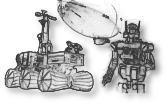## math/lapack

Linear Algebra PACKage.
Directory:
math/lapack (package's history)
Package version:
lapack-3.7.0r1
http://www.netlib.org/lapack/index.html
modified-bsd
Source archive:
http://netlib.org/lapack/lapack-3.7.0.tgz
Description:
LAPACK is a highly portable Fortran 77 library which provides routines for solving systems of simultaneous linear equations, least-squares solutions of linear systems of equations, eigenvalue problems, and singular value problems. The associated matrix factorizations (LU, Cholesky, QR, SVD, Schur, generalized Schur) are also provided, as are related computations such as reordering of the Schur factorizations and estimating condition numbers. Dense and banded matrices are handled, but not general sparse matrices. In all areas, similar functionality is provided for real and complex matrices, in both single and double precision.
Run dependencies:
gcc-fortran>=3
Build dependencies:
cmake>=2.6, digest>=20080510, patch>=2.0, pax, pkg_install>=20110805.12, tnftp>=20091122
fortran-compiler alternatives:
gfortran
Use the GNU fortran compiler
g95
Use the g95 fortran compiler